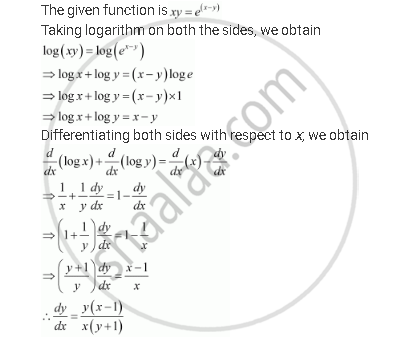Share

# Find Dy/Dx of Function Xy = E(X – Y) - CBSE (Commerce) Class 12 - Mathematics

#### Question

Find dy/dx of function

xy = e(x – y)

#### SolutionIs there an error in this question or solution?

#### APPEARS IN

NCERT Solution for Mathematics Textbook for Class 12 (2018 to Current)
Chapter 5: Continuity and Differentiability
Q: 15 | Page no. 178

#### Video TutorialsVIEW ALL 

Solution Find Dy/Dx of Function Xy = E(X – Y) Concept: Logarithmic Differentiation.
S